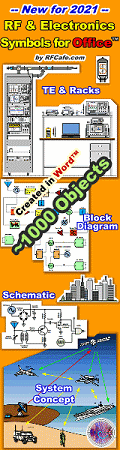Left Border Content - RF CafeAbout RF CafeCopyright: 1996 - 2024Webmaster:    Kirt Blattenberger,     BSEE - KB3UON RF Cafe began life in 1996 as "RF Tools" in an AOL screen name web space totaling 2 MB. Its primary purpose was to provide me with ready access to commonly needed formulas and reference material while performing my work as an RF system and circuit design engineer. The World Wide Web (Internet) was largely an unknown entity at the time and bandwidth was a scarce commodity. Dial-up modems blazed along at 14.4 kbps while typing up your telephone line, and a nice lady's voice announced "You've Got Mail" when a new message arrived... All trademarks, copyrights, patents, and other rights of ownership to images and text used on the RF Cafe website are hereby acknowledged. My Hobby Website: AirplanesAndRockets.comSub-Header - RF Cafe# Module 13 - Introduction to Number Systems and LogicNavy Electricity and Electronics Training Series (NEETS)Chapter 3:  Pages 3-41 through 3-46

Module 13 - Introduction to Number Systems and LogicFigure 3-36. - Integrated logic circuits: A. Shift register; B. Logic package.

Q61.   What are RTL, DTL, and TTL examples of?

Q62.   What type of logic family uses diodes in the input?

Q63.   What is the most common type of integrated circuit packaging found in military equipment?

Q64.   Circuits that can be interconnected without additional circuitry are known as..................     circuits.

SUMMARY

Now that you have completed this chapter, you should have a basic understanding of the more common special logic circuits. The following is a summary of the emphasized terms and points found in the "Special Logic Circuits" chapter.

SPECIAL LOGIC CIRCUITS perform arithmetic and logic operations; input, output, store and transfer information; and provide proper timing for these operations.

EXCLUSIVE OR (X-OR) circuits produce a 1 output when ONLY one input is HIGH. Can be used as a quarter adder.

3-41EXCLUSIVE NOR (X-NOR) circuits produce a 1 output when all inputs are 0 and when more than 1 input is 1.QUARTER ADDER circuits produce the sum of two numbers but do not generate a carry.

HALF ADDER circuits produce the sum of two numbers and generate a carry.STANDARD SYMBOLS depict logic circuitry with blocks, showing only inputs and outputs. One block may contain many types of gates and circuits.

SUBTRACTION in binary is accomplished by complementing and adding.

FLIP-FLOP are bistable multivibrators used for storage, timing, arithmetic operations, and transfer of information.

R-S FFs have the Q output of the FF HIGH in the set mode and LOW in the reset mode.3-42

T (TOGGLE) FFS  change state with each pulse applied to the input. Each T FF will divide the input by 2.D FF is used to store data at a predetermined time.J-K FF is the most versatile FF. J-Ks can perform the same functions as all the other FFs.CLOCKS are circuits that generate the timing and control signals for other operations.

COUNTERS are used to count operations, quantities, or periods of time. They can be used to divide frequencies, to address information in storage, or as temporary storage.

MODULUS of a counter is the total number of counts or stable states a counter may indicate.

UP COUNTERS count from 0 to a predetermined number.

DOWN COUNTERS count from a predetermined number to 0.

RING COUNTERS are loop counters that may be used for timing operations.

REGISTERS are used as temporary storage devices as well as for transfer of information.

PARALLEL REGISTERS receive or transfer all bits of data simultaneously.

SHIFT REGISTERS are used to perform serial-to-parallel and parallel-to-serial conversion and for scaling binary numbers.

3-43

SERIAL TRANSFER causes all bits of data to be transferred on a single line.

PARALLEL TRANSFER has each data bit on its own line.

SCALING of binary numbers means to increase or decrease the magnitude of a number by a power of 2.

LOGIC FAMILIES are composed of logic circuits based on particular types of elements.

ANSWERS TO QUESTIONS Q1. THROUGH Q64.

A1.   .

A2.   Low (0).

A3.   One or the other of the inputs must be HIGH, but not both at the same time.

A4.   Exclusive NOR (X-NOR).

A5.   HIGH.

A6.   The half adder generates a carry.

A8.   Sum equals 0 with a carry of 1.

A10.   Four.

A11.   S1 = 1, S2  = 0 and C2 = 1.

A12.   C1 = 0.

A13.   X-OR gates.

A14.   Four.

A15.   MSD of the sum.

A17.   Subtrahend.

A18.   Storing information.

A19.   Six.

A20.   1 and 0, or opposite states.

A21.   By cross-coupling NAND or OR gates.

A22.   One.

3-44

A23.   To divide the input by 2.

A24.   Clock and data.

A25.   Up to one clock pulse.

A26.   A positive-going clock pulse.

A27.   J-K flip-flop.

A28.   Set, or HIGH (1).

A29.   When the clock pulse goes LOW.

A30.   Both J and K must be HIGH.

A31.   Clear (CLR) and preset (PS or PR).

A32.   The flip-flop is jammed.

A33.   A timing signal.

A34.   An astable or free-running multivibrator.

A35.   Triggers.

A36.   A multiphase clock.

A37.   32.

A38.   Ripple.

A39.   Toggle.

A40.   Synchronous.

A41.   The AND gate.

A42.   11112, or 1510.

A43.   Four.

A44.   FFs 2 and 4.

A45.   Two.

A46.   Three.

A47.   The input, or clock pulse.

A48.   One.

A49.   Four.

A50.   Q output of FF 1 going LOW.

3-45

A51.   16.

A52.   Parallel.

A53.   By clearing the register.

A54.   Shift register.

A55.   Serial.

A56.   Requires more circuitry.

A57.   Eight.

A58.   Four.

A59.   22, or four times.

A60.   Three to the left.

A61.   Logic families.

A62.   DTL (diode transistor logic).

A63.   DIPs (dual inline packages).

A64.   Compatible.

3-46

Footer - RF CafePlease Support RF Cafe by purchasing my  ridiculously low−priced products, all of which I created. These Are Available for Free